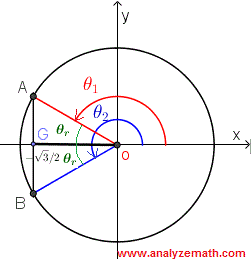# Solve Trigonometric Equations Examples and Questions With Solutions

How to solve trigonometric equations? Questions are presented along with detailed solutions and explanations.

## How to Solve Trigonometric Functions?

### Example 1

Find all the solutions of the trigonometric equation √3 sec(θ) + 2 = 0
Solution:
Using the identity sec(θ) = 1 / cos(θ), we rewrite the equation in the form
cos(θ) = - √3 / 2
Find the reference θr angle by solving cos(θr) = √3 / 2 for θr acute.
θr = π/6
Use the reference angle θr to determine the solutions θ1 and θ2 on the interval [0 , 2π) of the given equation. The equation cos(θ) = - √3 / 2 suggests that cos(θ) is negative and that means the terminal side of angle θ solution to the given equation is either in quadrants II or III as shown below using the unit circle.Hence the solutions:
θ1 = π - θr = π - π/6 = 5π/6
θ2 = π + θr = π + π/6 = 7π/6
Use the solutions on the interval [0 , 2π) to find all solutions by adding multiples of 2π as follows:
θ1 = 5π/6 + 2nπ , n = 0, ~+mn~ 1 , ~+mn~ 2, ...
θ2 = 7π/6 + 2nπ , n = 0, ~+mn~ 1 , ~+mn~ 2, ...
Below are shown the graphical solutions on the interval [0 , 2π).

### Example 2

Solve the trigonometric equation 2 sin(θ) = -1
Solution:
Rewrite the above equation in simple form as shown below.
sin(θ) = -1/2
Find the reference θr angle by solving sin(θ) = 1/2 for θr acute.
θr = π/6
Use the reference angle θr to determine the solutions θ1 and θ2 on the interval [0 , 2π) of the given equation. The equation sin(θ) = - 1 / 2 suggests that sin(θ) is negative and that means the terminal side of angle θ is either in quadrants III or VI as shown in the unit circle below.Hence the solutions:
θ1 = π + θr = 7π/6
θ2 = 2π - θr = 11π/6
Use the solutions on the interval [0 , 2π) to find all solutions by adding multiples of 2π as follows:
θ1 = 7π/6 + 2nπ , n = 0, ~+mn~ 1 , ~+mn~ 2, ...
θ2 = 11π/6 + 2nπ , n = 0, ~+mn~ 1 , ~+mn~ 2, ....

### Example 3

Solve the trigonometric equation √2 cos(3x + π/4) = - 1
Solution:
Let θ = 3x + π/4 and rewrite the equation in simple form.
√2 cos(θ) = - 1
cos(θ) = -1/√2
Find the reference θr angle by solving cos(θ) = 1/√2 for θr acute.
θr = π/4
Use the reference angle θr to determine the solutions θ1 and θ2 on the interval [0 , 2π) of the given equation. The equation cos(θ) = - 1/√2 suggests that cos(θ) is negative and that means the terminal side of angle θ is either in quadrants II or III. Hence the two solutions of the equation cos(θ) = - 1/√2 on the interval [0 , 2π) are given by
θ1 = π - θr = 3π/4
θ2 = π + θr = 5π/4
We now write the general solutions by adding multiples of 2π as follows:
θ1 = 3π/4 + 2nπ , n = 0, ~+mn~ 1 , ~+mn~ 2, ...
θ2 = 5π/4 + 2nπ , n = 0, ~+mn~ 1 , ~+mn~ 2, ...
We now substitute θ1 and θ2 by the expression 3x + π/4
3x + π/4 = 3π/4 + 2nπ
3x + π/4 = 5π/4 + 2nπ
and solve for x to obtain the solutions for x.
x = π/6 + 2nπ/3 , n = 0, ~+mn~ 1 , ~+mn~ 2, ...
x = π/3 + 2nπ/3 , n = 0, ~+mn~ 1 , ~+mn~ 2, ...

### Example 4

Solve the trigonometric equation - 2 sin 2x - cos x = - 1
Solution:
The above equation may be factored if all trigonometric functions included in that equation are the same. So using the identity sin 2x = 1 - cos 2x, we can rewrite the above equation using the same trigonometric function cos x as follows:
- 2 (1 - cos
2x) - cos x = - 1
Simplify and rewrite as
2 cos
2x - cos x - 1 = 0
Factor the left hand side
(2 cos x + 1)(cos x - 1) = 0
Hence the two equations to solve
(1) 2 cos x + 1 = 0 and (2) cos x - 1 = 0
Solve equation (1) using the reference angle as was done in the examples above.
cos x = -1/2
x
1 = 2π/3 + 2nπ , n = 0, ~+mn~ 1 , ~+mn~ 2, ...
x
2 = 4π/3 + 2nπ , n = 0, ~+mn~ 1 , ~+mn~ 2, ...
Solve equation (2)
cos x = 1
x
3 = 2nπ , n = 0, ~+mn~ 1 , ~+mn~ 2, ...